一种非最小耦合暗能量模型的研究Study on a Kind of Non-Minimal Coupling Dark Energy Models

• 全文下载: PDF(1044KB)    PP.284-289   DOI: 10.12677/MP.2018.86032
• 下载量: 250  浏览量: 609

This paper studies a kind of non-minimum coupling dark energy models. In the dark energy models, the coupling form of the dark energy as a scalar field and gravity is  F(∅)=1+α∅2. We studied the characteristics and evolution behavior of the dark energy field, and analyzed the influence of different parameters on the models. Through analysis, it is found that the parameter α plays a key role in the evolution of the model—whether the dark energy always appears as a Quintessence field.

1. 引言

2. 一般形式的非最小耦合暗能量模型

$L=\frac{F\left(\varphi \right)R}{16\text{π}G}+\frac{1}{2}k\left(\varphi \right){\nabla }_{\mu }\varphi {\nabla }^{\mu }\varphi -V\left(\varphi \right)+{L}_{m}$ (1)

$S={\int }^{\text{​}}{\text{d}}^{4}x\sqrt{-g}\left[\frac{F\left(\varphi \right)R}{16\text{π}G}+\frac{1}{2}k\left(\varphi \right){\nabla }_{\mu }\varphi {\nabla }^{\mu }\varphi -V\left(\varphi \right)+{L}_{m}\right]$ (2)

${\nabla }_{\mu }{\nabla }^{\mu }\varphi =-\frac{1}{k\left(\varphi \right)}\left[\frac{-{F}^{\prime }\left(\varphi \right)R}{16\text{π}G}+\frac{1}{2}{k}^{\prime }\left(\varphi \right){\nabla }_{\mu }\varphi {\nabla }^{\mu }\varphi +{V}^{\prime }\left(\varphi \right)\right]$ (3)

$\frac{F\left(\varphi \right)}{16\text{π}G}\left({R}^{\mu \nu }-\frac{1}{2}{g}^{\mu \upsilon }R\right)=-\frac{1}{2}{T}_{m}^{\mu \nu }-\frac{1}{2}{T}_{\varphi }^{\mu \nu }+\frac{1}{16\text{π}G}\left[{\nabla }_{\mu }{\nabla }^{\mu }F\left(\varphi \right){g}^{\mu \upsilon }-F{\left(\varphi \right)}^{;\mu ;\nu }\right]$ (4)

${T}_{m}^{\mu \nu }$ 为物质和辐射的能动量张量， ${T}_{\varphi }^{\mu \nu }$ 表示暗能量的能动量张量，即：

${T}_{\varphi }^{\mu \nu }=k\left(\varphi \right)\left({\nabla }^{\mu }\varphi {\nabla }^{\nu }\varphi -\frac{1}{2}{g}^{\mu \nu }{\nabla }_{\mu }\varphi {\nabla }^{\mu }\varphi \right)+V\left(\varphi \right){g}^{\mu \upsilon }$

$R=8\text{π}G\frac{-k\left(\varphi \right){\nabla }_{\mu }\varphi {\nabla }^{\mu }\varphi +4V\left(\varphi \right)+{\rho }_{m}-3{P}_{m}-3{\nabla }_{\mu }{\nabla }^{\mu }F\left(\varphi \right)}{F\left(\varphi \right)}$ (5)

$\begin{array}{l}{\nabla }_{\mu }{\nabla }^{\mu }\varphi =-\frac{1}{k\left(\varphi \right)}\left[\frac{-{F}^{\prime }\left(\varphi \right)\left(8\text{π}G\frac{-k\left(\varphi \right){\nabla }_{\mu }\varphi {\nabla }^{\mu }\varphi +4V\left(\varphi \right)+{\rho }_{m}-3{P}_{m}-3{\nabla }_{\mu }{\nabla }^{\mu }F\left(\varphi \right)}{F\left(\varphi \right)}\right)}{16\text{π}G}\\ \text{\hspace{0.17em}}\text{\hspace{0.17em}}\text{\hspace{0.17em}}\text{\hspace{0.17em}}\text{\hspace{0.17em}}\text{\hspace{0.17em}}\text{\hspace{0.17em}}\text{\hspace{0.17em}}\text{\hspace{0.17em}}\text{\hspace{0.17em}}\text{ }\begin{array}{c}\underset{}{\overset{}{}}\\ \\ \end{array}+\frac{1}{2}{k}^{\prime }\left(\varphi \right){\nabla }_{\mu }\varphi {\nabla }^{\mu }\varphi +{V}^{\prime }\left(\varphi \right)\right]\end{array}$ (6)

3. 具体形式为 $F\left(\varphi \right)=1+a{\varphi }^{2}$$K\left(\varphi \right)=1$ 的暗能量模型

$S={\int }^{\text{​}}{\text{d}}^{4}x\sqrt{-g}\left[\frac{1+a{\varphi }^{2}R}{16\text{π}G}+\frac{1}{2}{\nabla }_{\mu }\varphi {\nabla }^{\mu }\varphi -V\left(\varphi \right)+{L}_{m}\right]$ (7)

$\rho =\frac{\stackrel{˙}{\varphi }}{2}+3a{H}^{2}{\varphi }^{2}+V\left(\varphi \right)-6aH\varphi \stackrel{˙}{\varphi }$ (8)

$P=\frac{\stackrel{˙}{\varphi }}{2}-a\left(2\stackrel{˙}{H}+3{H}^{2}\right){\varphi }^{2}-V\left(\varphi \right)-4aH\varphi \stackrel{˙}{\varphi }-2a\varphi \stackrel{¨}{\varphi }-2a{\stackrel{˙}{\varphi }}^{2}$ (9)

${w}_{DE}=\frac{{P}_{DE}}{{\rho }_{DE}}=\frac{\frac{\stackrel{˙}{\varphi }}{2}-a\left(2\stackrel{˙}{H}+3{H}^{2}\right){\varphi }^{2}-V\left(\varphi \right)-4aH\varphi \stackrel{˙}{\varphi }-2a\varphi \stackrel{¨}{\varphi }-2a{\stackrel{˙}{\varphi }}^{2}}{\frac{\stackrel{˙}{\varphi }}{2}+3a{H}^{2}{\varphi }^{2}+V\left(\varphi \right)-6aH\varphi \stackrel{˙}{\varphi }}$ (10)

4. 模型演化两种不同情况的分析

1) 耦合系数a > 0

${\text{Ω}}_{m}=\frac{{\rho }_{matt}+{\rho }_{rad}}{\frac{{\omega }_{0}{\stackrel{˙}{\varphi }}^{2}}{\varphi }+V\left(\varphi \right)-3H\stackrel{˙}{\varphi }+{\rho }_{matt}+{\rho }_{rad}}\approx 30%$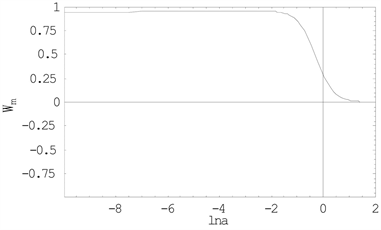Figure 1. Percentage of matter and radiation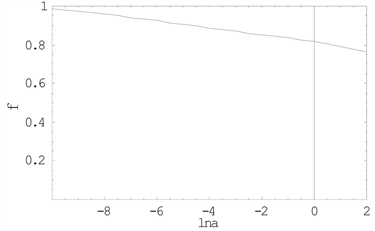Figure 2. The evolution of $\varphi$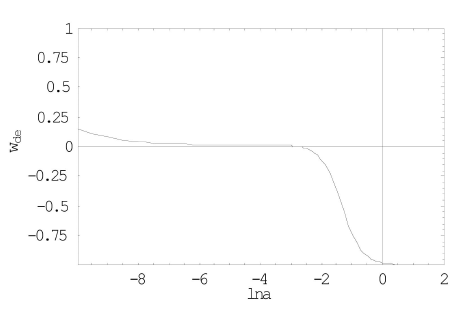Figure 3. The state equation w of the dark energy

2) 耦合系数a < 0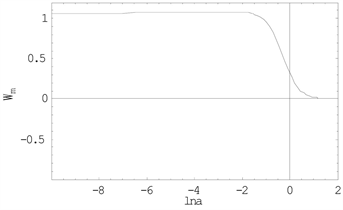Figure 4. Percentage of matter and radiation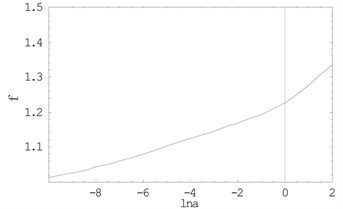Figure 5. The evolution of $\varphi$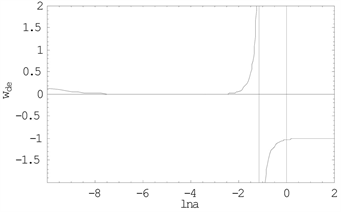Figure 6. The state equation w of the dark energy

5. 结论

  Riess, A.G., et al., Supernova Search Team Collaboration. (1998) Observational Evidence from Supernovae for an Accelerating Universe and a Cosmological Constant. Astronomical Journal, 116, 1009-1038. https://doi.org/10.1086/300499  Perlmutter, S., et al., Supernova Cosmology Project Collaboration. (1999) Measurements of Omega and Lambda from 42 High Redshift Supernovae. Astronomical Journal, 517, 565-586. https://doi.org/10.1086/307221  Tonry, J.L., et al., Supernova Search Team Collaboration. (2003) Cosmo-logical Results from High-z Supernovae. Astronomical Journal, 594, 1-24.  Riess, A.G., et al., Supernova Search Team Collaboration. (2004) Type Ia Supernova Discoveries at z > 1 from the Hubble Space Telescope: Evidence for Past Deceleration and Constraints on Dark Energy Evolution. Astronomical Journal, 607, 665-687. https://doi.org/10.1086/383612  Clocchiatti, A., et al., The High Z SN Search Collaboration. (2006) Hubble Space Telescope and Ground-Based Observations of Type Ia Supernovae at Redshift 0.5: Cosmological Implications. Astronomical Journal, 642, 1-21.  Di Valentino, E. and Melchiorri, A. (2017) First Cosmological Constraints Combining Planck with the Recent Gravitational-Wave Standard Siren Measurement of the Hubble Constant. e-Print: arXiv:1710.06370.  Ooba, P., Ratra, B. and Sugiyama, N. (2018) Planck 2015 Constrains on Spatially-Flat Dy-namical Dark Energy Models. Arxiv:1802.05571v1[astro-ph.CO].  Aghanim, N., et al. (2018) Planck 2018 Results. VI. Cosmological Parameters. Arxiv:1807:06209V1[astro-ph.CO].  Berti, E., et al. (2015) Testing General Relativity with Present and Future Astrophysical Observations. Classical and Quantum Gravity, 32, 243001-243179. https://doi.org/10.1088/0264-9381/32/24/243001  Ooba, J., Ichiki, K., Chiba, T. and Sugiyama, N. (2017) Cosmological Constraints on Scalar-Tensor Gravity and the Variation of the Gravitational Constant. Progress of Theo-retical and Experimental Physics, No. 4, 043E03-043E18. https://doi.org/10.1093/ptep/ptx046  Zhang, X.-F. (2017) Evolution of the Universe in Scalar-Tensor Gravity. Modern Physics, 7, 242-248. https://doi.org/10.12677/MP.2017.76028  Zhang, X.-F. (2011) Dark Energy Model with Non-Minimal Coupling and Cosmological Constant Boundary. Communications in Theoretical Physics, 56, 1167-1170. https://doi.org/10.1088/0253-6102/56/6/34  Torres, D.F. (2002) Quintessence, Super Quintessence and Ob-servable Quantities in Brans-Dicke and Non-Minimally Coupled Theories. Physics Review, D66, Article ID: 043522. https://doi.org/10.1103/PhysRevD.66.043522